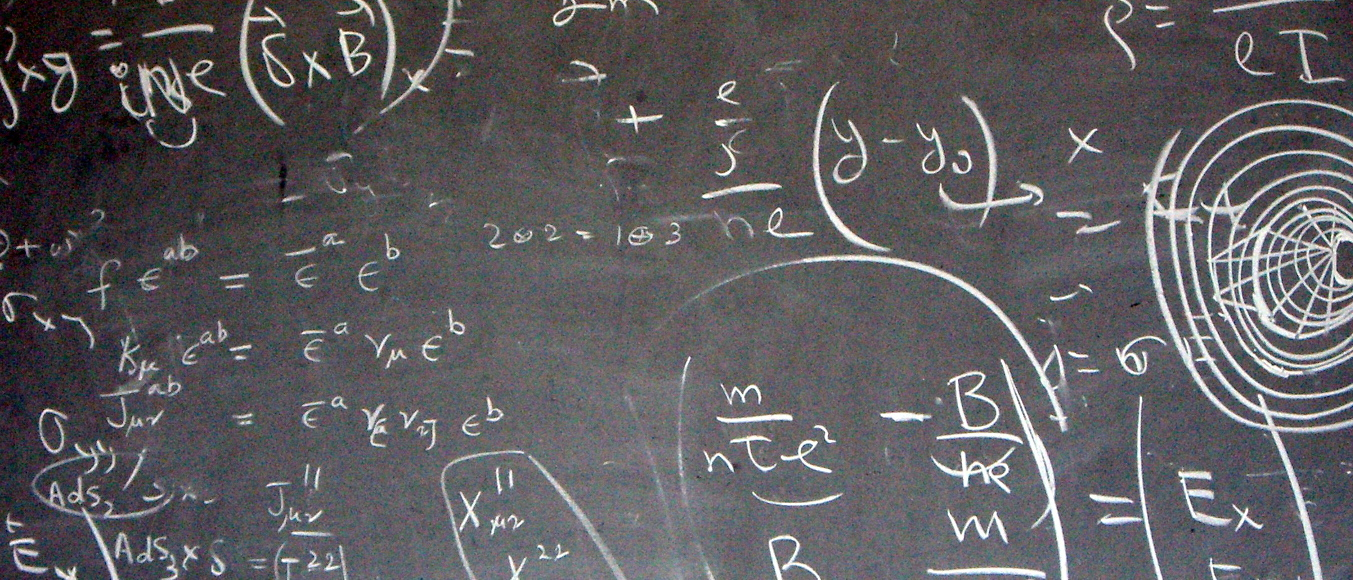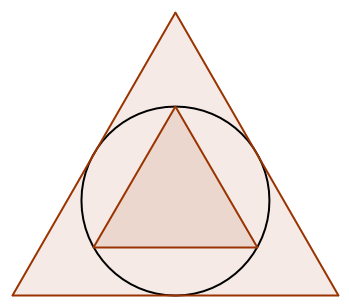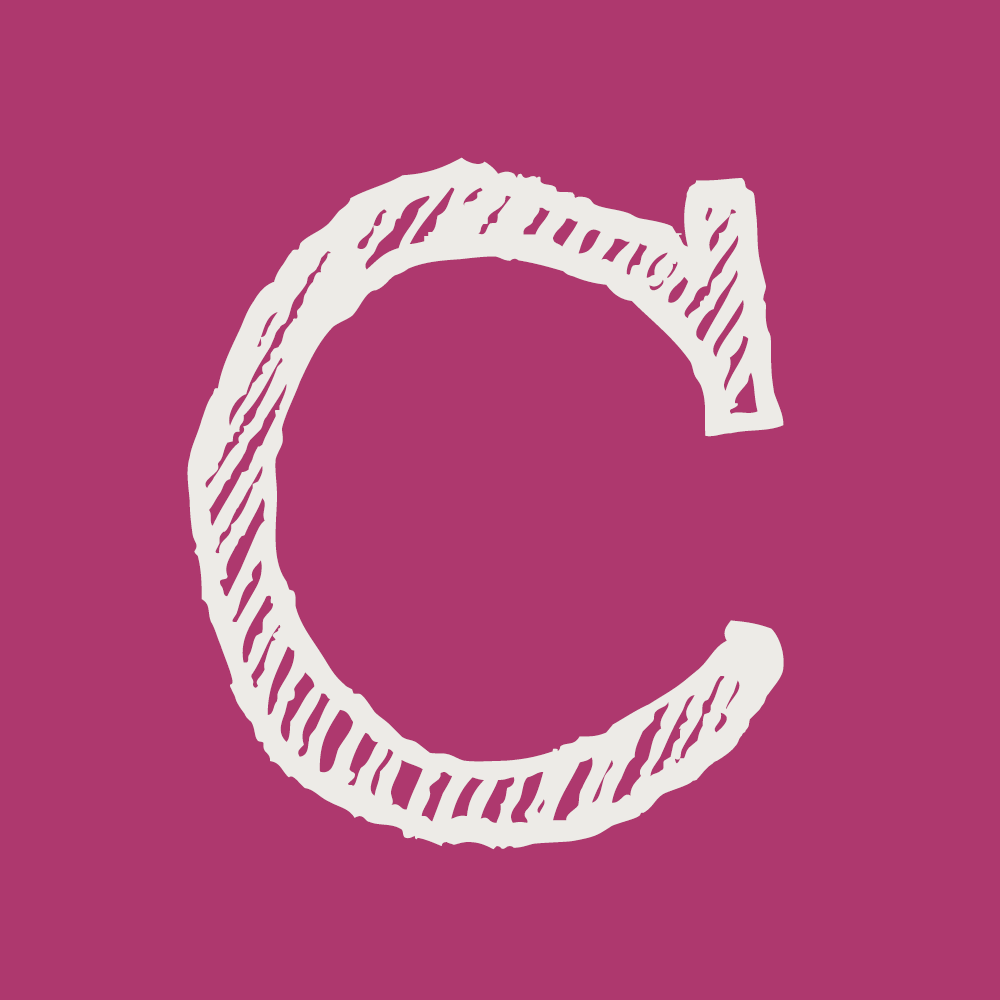# What makes a good puzzle?

If you enjoyed Hannah’s sweets then you’ll love these…Recently, mathematical puzzles have been hitting the headlines. First, there was the Vietnamese problem for eight-year-olds, which proved too difficult for many adults. Then Hannah’s Sweets, a question from this year’s GCSE exam, spread through Twitter like wildfire, leaving teenagers weeping in its wake.

Perhaps surprisingly to those teenagers, many people work on this sort of puzzle for fun. But what is it that makes a good puzzle?

### Surprise

One thing that makes a puzzle memorable is a surprise: often a familiar answer appearing in an unexpected place. Polya Strikes Out is one puzzle that leads to a surprisingly familiar sequence.

### Polya Strikes Out

Source: Thinking Mathematically by John Mason, Leone Burton & Kaye Stacey
Write the numbers 1, 2, 3, … in a row. Strike out every third number beginning with the third. Write down the cumulative sums of what remains:
1, 2, 3, 4, 5, 6, 7, …
1, 2, 3, 4, 5, 6, 7, …
1, 2, 4, 5, 7, …
1=1; 1+2=3; 1+2+4=7; 1+2+4+5=12; 1+2+4+5+7=19; …
1, 3, 7, 12, 19, …
Now strike out every second number beginning with the second. Write down the cumulative sums of what remains.
What is the final sequence?
Why do you get this sequence?

Another cause of surprise is a seemingly impossible situation, or a paradox. The following puzzle contains an impossibility. Can you find the mistake in its deduction?

### Every Number Is Greater Than Itself

Source: Riddles in Mathematics by Eugene P. Northrop
Let $a$ and $b$ be two positive numbers such that $a>b$.
Multiplying both sides by $b$ gives $$ab>b^2.$$ Subtracting $a^2$ from both sides then factorising gives $$a(b-a)>(b+a)(b-a).$$ Dividing both sides by $b-a$ gives $$a>b+a.$$ Since $b$ is positive, $a$ is bigger than itself and bigger than every number larger than itself.
This is obviously incorrect. Where is the mistake?

### Insight

Good puzzles often lead to an insight, or a different way of thinking about a situation. Here are two puzzles whose solutions require finding such an insight.

### The Mutiliated Chessboard

Source: My Best Mathematical and Logic Puzzles by Martin Gardner
A normal chessboard can be covered by 32 dominos (with each domino the right size to cover two squares).
Two squares at the opposite corners of a chessboard have been cut off. Is it possible to cover this chessboard with 31 dominos?

### Two TrianglesThe diagram shows a circle and two equilateral triangles: one whose edges are tangent to the circle and one whose vertices lie on the circle. What is the area of the smaller triangle as a fraction of the area of the larger triangle?

### Open Endedness

Another feature that makes puzzles memorable is an open ending that leads to further questions and discoveries, like the following puzzles:

### Bouncing Ball

Source: Starting Points by C.S. Banwell, K.D. Saunders & D.G.Tahta
In a 4×3 rectangle, a ball is fired from the top left corner at 45°.It bounces around a rectangle until it hits a corner. Which corner does it end in?
Which corner will it end in for rectangles of other sizes?

### Reverse Bases

Find two digits $a$ and $b$ such that $ab$ in base 10 is equal to $ba$ in base 4.
Find two digits $c$ and $d$ such that $cd$ in base 10 is equal to $dc$ in base 7.
Find two digits $e$ and $f$ such that $ef$ in base 9 is equal to $fe$ in base 5.

For which pairs of bases $A$ and $B$ can you find two digits $g$ and $h$ such that $gh$ in base $A$ is equal to $hg$ in base $B$?Matthew Scroggs is a postdoctoral researcher in the Department of Engineering at the University of Cambridge working on finite and boundary element methods. His website, mscroggs.co.uk, is full of maths.
@mscroggs    mscroggs.co.uk    + More articles by Matthew

## One thought on “What makes a good puzzle?”

1.Earle Jones says:

y = x^x

What does this simple dependence look like in the left-half plane?

Hint: x = -1/3 makes y real.
x = -1/2 makes y imaginary.